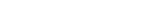1388 is valid Arabic numeral. Here we will explain how to read, write and convert the Arabic numeral 1388 into the correct Roman numeral format. Please have a look over the Roman numeral table given below for better understanding of Roman numeral system. As you can see, each letter is associated with specific value.

Symbol Value
I1
V5
X10
L50
C100
D500
M1000

# How to write Arabic Numeral 1388 in Roman numeral?

The Roman numeral representation of Arabic numeral 1388 is MCCCLXXXVIII.

# How to convert Arabic numeral 1388 to Roman numeral?

If you are aware of Roman numeral system, then converting Arabic numeral 1388 to Roman numeral is very easy. Converting 1388 to Roman numeral representation involves breaking up the numeral into place values as shown below.

 1388 1000 + 100 + 100 + 100 + 50 + 10 + 10 + 10 + 5 + 1 + 1 + 1 M + C + C + C + L + X + X + X + V + I + I + I MCCCLXXXVIII

We need to combine all converted roman numerals together. As per the rule highest numeral should always precede the lowest numeral to get correct representation. In modern world, the number 1388 should be used as it is until or unless it represents an ordinal value. In case of any ordinal value, you can use MCCCLXXXVIII instead of 1388. For any numeral conversion, you can also use our roman to number converter tool given above.

Disclaimer:We make a reasonable effort in making sure that conversion results are as accurate as possible, but we cannot guarantee that. Before using any details provided here, you must validate its correctness from other reliable sources on internet.